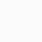Maths And Physics With Pandey Sir Ncert 12th Physics Chapter-12 α-Particle Scattering Experiment by Rutherford

# α-Particle Scattering Experiment by Rutherford

In this experiment H Geiger and E Marsden( Student of Rutherford and on suggestion of Rutherford) took ₈₃Bi²¹⁴ (Bismuth) as a source of α particles. A collimated beam of α particles of energy range (5 MeV to 7.7 MeV) was allowed to fall on 10⁻⁸ m to 10⁻⁶ m thick gold foil. The α particles were observed through a rotatable detector consisting of a zinc sulphide screen and microscope and it was found that α particle got scattered. These scattered α particle produced scintillations on the zinc sulphide screen. Now these scintillations were are counted at different angles from the direction of incident beam(say θ).

Observations:

(I) Most of α particles passed through the gold foil without any deflection or in straight line (approx 99.86 %).

(II) Only about 0.14 % of the incident α particle scattering by more than 1⁰

(III) About 1 α particle in every 8000 α particles deflected by more than 90⁰.

(IV) About 1 in 10⁵ α particles deflected by 180⁰ and returns back.

(V) The number of particles scattered at angle θ are given by

N= kz²/Sin⁴(θ/2)

Where, N = Number of α particle
k = Constant
Z = Atomic number of atom
θ = Scattering angle

(VI) The graph between total number of α particle(N) and scattered through at angle θ is (Experimentally)

From the above experiment Rutherford concludes the following.

Rutherford Nuclear Model of Atom:

1. Since most α particles passes undeviated that means atom has a lot of empty space and practically the entire mass of the atom is confined to an extremely small central core called nucleus, whose size is of the order of 10⁻¹⁵ to 10⁻¹⁴ m.

2. Scattering of α particles(+Ve) is due to the Coulomb’s law for electrostatic force of repulsion between the positive charge of the nucleus and α particles.

3. Distance between electron and nucleus is from 10⁴ to 10⁵ times the size of nucleus itself.

4. The size of nucleus is of order of 10⁻¹⁵ m, which is very small as compared to the size of the atom which is of the order of 10⁻¹⁴ m.

5. The atomic nucleus is surrounded by certain number of electron. The total number of negative charge of electrons surrounding the nucleus is equal to the total positive charge on the nucleus.

6. These electrons revolve around the nucleus in various circular orbits as the planets do around the sun.

The electrons are attracted by nucleus due to electrostatic force but are not pulled toward it as they are constantly revolving in circular path using this electrostatic force as centripetal force(fe=fc).

## 1 thought on “α-Particle Scattering Experiment by Rutherford”

1.Anonymous says:

nice